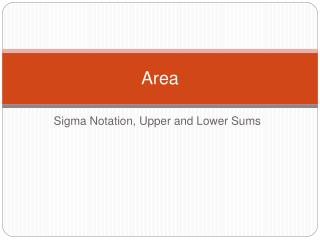Download PresentationArea

# Area - PowerPoint PPT PresentationDownload Presentation## Area

- - - - - - - - - - - - - - - - - - - - - - - - - - - E N D - - - - - - - - - - - - - - - - - - - - - - - - - - -
##### Presentation Transcript

1. Area Sigma Notation, Upper and Lower Sums

2. Sigma Notation • Definition – a concise notation for sums. • This notation is called sigma notation because it uses the uppercase Greek letter sigma, written as ∑. • The sum of n terms

3. Examples of Sigma Notation

4. Examples of Sigma Notation

5. Examples of Sigma Notation

6. Summation Formulas

7. Using Formulas to Evaluate a Sum • Evaluate the following summation for n = 10, 100, 1000 and 10,000.

8. Using Formulas to Evaluate a Sum • Now we have to substitute 10, 100, 1000, and 10,000 in for n. • n = 10 the answer is 0.65000 • n = 100 the answer is 0.51500 • n = 1000 the answer is 0.50150 • n = 10,000 the answer is 0. 50015 • What does the answer appear to approach as the n’s get larger and larger (limit as n approaches infinity)?

9. Area • Finding the area of a polygon is simple because any plane figure with edges can be broken into rectangles and triangles. • Finding the area of a circular object or curve is not so easy. • In order to find the area, we break the figure into rectangles. The more rectangles, the more accurate the area will be.

10. Approximating the Area of a Plane Region • Use five rectangles to find two approximations of the area of the region lying between the graph of • and the x-axis between the graph of x = 0 and x = 2.

11. Steps • 1. Draw the graph • 2. Find the width of each rectangle by taking the larger number and subtracting the smaller number. Then divide by the number of rectangles designated. • 3. Now find the height by putting the x values found in number 2 into the equation. • 4. Multiply the length times the height (to find the area of each rectangle). • 5. Add each of these together to find the total area.

12. Approximating the Area of a Plane Region

13. Approximating the Area of a Plane Region

14. Approximating the Area of a Plane Region • Now let’s find the area using the left endpoints. The five left endpoints will involve using the i – 1 rectangle. This answer will be too large because there is lots of area being counted that is not included (look at the graph).

15. Approximating the Area of a Plane Region

16. Approximating the Area of a Plane Region • The true area must be somewhere between these two numbers. • The area would be more accurate if we used more rectangles. • Let’s use the program from yesterday to find the area using 10 rectangles, 100 rectangles, and 1000 rectangles. • What do you think the true area is?

17. Upper and Lower Sums • An inscribed rectangle lies inside the ith region • A circumscribed rectangle lies outside the ith region • An area found using an inscribed rectangle is smaller than the actual area • An area found using a circumscribed rectangle is larger than the actual area • The sum of the areas of the inscribed rectangles is called a lower sum. • The sum of the areas of the circumscribed rectangles is called an upper sum.

18. Example of Finding Upper and Lower Sums • Find the upper an lower sums for the region bounded by the graph of • Remember to first draw the graph. • Next find the width using the formula

19. Example of Finding Lower and Upper Sums

20. Example of Finding Lower Sum

21. Example of Finding Lower Sum

22. Finding an Upper Sum • Using right endpoints

23. Limit of the Lower and Upper Sums • Let f be continuous and nonnegative on the interval • [a, b]. The limits as n —›∞ of both upper and lower sums exist and are equal to each other. That is,

24. Definition of the Area of a Region in the Plane • Let f be continuous and nonnegative on the interval • [a, b]. The area of the region bounded by the graph of f, the x-axis, and the vertical lines x = a and x = b is